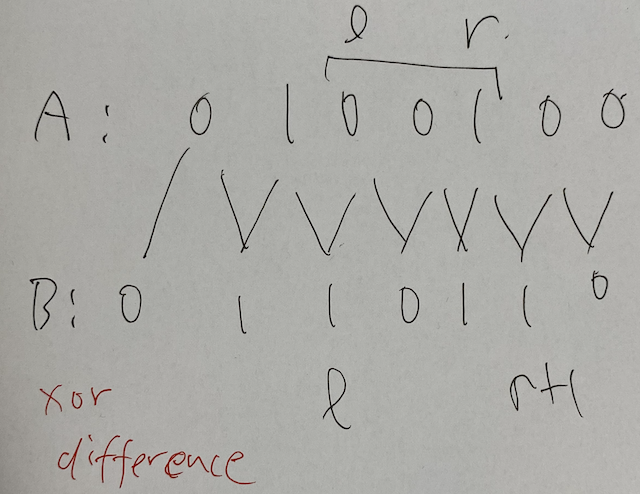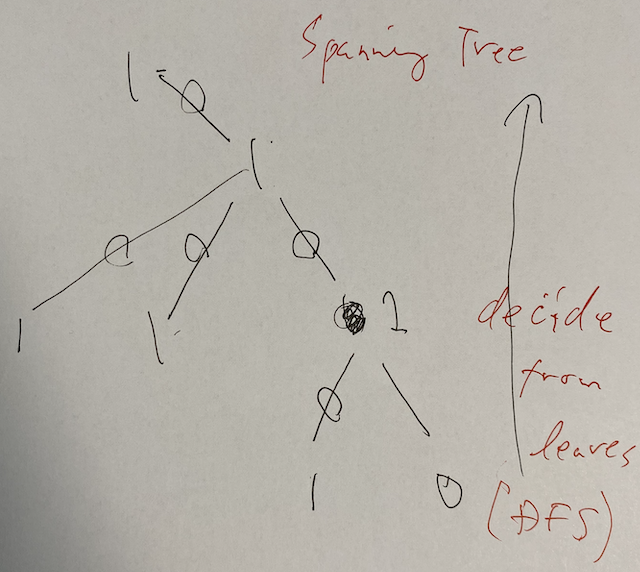# AtCoder Beginner Contest 155

Updated:

AtCoder Beginner Contest 155

• Review: 2020-06-17

# Solutions

## A - Poor

We get the input by vector<int> A(3);. We sort it. The answer is Yes if either of the following holds.

• $A = A$ and $A \neq A$,
• $A \neq A$ and $A = A$.

If we find such $A$ that $A \% 2 = 0 \land A \% 3 \neq 0 \land A \% 5 \neq 0,$ the answer is No. Otherwise, Yes.

## C - Poll

We define map<string, int> M; and we gather the poll papers. Then, we get the maximum of the number of votes. We again search $M$ for the strings that are written on the maximal number and flush them. They are automatically in lexicographical order.

## D - Pairs

### Observation

The answer is the maximum value of $X$ so that there is less than $K$ pairs whose product is less than $X$. This is a problem for us to use binary search for $X$.

We divide this problem into three cases.

Therefore, we split $A$ into there vectors $plus$, $zero$, $minus$. We can ignore $zero$. Let $P = \lvert plus \rvert$ and $M = \lvert minus \rvert$. Let $S _ p$, $S _ z$ and $S _ m$ be the numbers of the positive, zero, and negative pairs, respectively. We see that $S _ p = P (P - 1) / 2 + M (M - 1) / 2$, $S _ m = PM$ and $S _ z = N (N - 1) / 2 - S _ p - S _ m$. Then, we now know the division into cases as follows.

1. The answer is negative if $K \leq S _ m$.
2. The answer is zero if neither 1. or 3. holds. In this case, flush $0$ immediately.
3. The answer is positive if $K > S _ m + S _ z$. In this case, we fix $K \gets K - (S _ m + S _ z)$.

### Solution

#### Case: Negative

We solve the case 1. We are going to solve this problem by binary search for the answer. We need a judge function.

$count(T) =$ the number of the pairs whose product is less than $T$.

This is the sum of the following function with $x \in minus$.

$count(T, x) =$ the number of the elements $y \in plus$ so that $xy$ is less than $T$.

We sort $plus$ in descending order and $minus$ in ascending order in advance. We can compute this function by binary search for the index of $plus$.

#### Case: Positive

Similar solution works for the case 3. We sort $plus$ in ascending order and $minus$ in descending order in advance. We mend the solution above by the following function.

$count(T, i, V) =$ the number of the elements $V[j]$ with $j > i$ so that $V[i]V[j]$ is less than $T$.

### Time complexity

The total time complexity will be $O((\log L) N \log N)$, where $L$ is the possible maximum value for this problem.

## E - Payment

### Observation

Suppose that we pay for “…3”. How many coins of $1$-denomination are used?

If both the customer and the shop uses at least one $1$-denomination, it is not the best strategy since they both reduce at least one $1$-denomination.

Hence, there are two possibilities for each digit.

• The customer uses the denomination and the shop won’t pay back.
• The customer does not use the denomination and the shop pay back.

For the former case, the customer uses three $1$-denomination. For the latter case, the shop pay back seven $1$-denomination. In this case, the customer will use one more $10$-denomination. Thus, in the digit-DP, we possess this state as well.

### Solution: Digit-DP

First, we reverse the string and let $N$ be the length of it.

#### Definition

$dp[i][j] =$ the minimum number of the coins considering $S[0, i)$. Here, $j = 0$ means that there is no moving up; $j = 1$ means that there is.

#### Initial state

$dp = 1$. Otherwise, $\infty$.

$\min(dp[N], dp[N] + 1)$.

The latter case occurs when, for example, we pay $9,999$ yen and we use one $10,000$-yen bill.

#### Transition

We will mean by $k = 0$ that we will resolve moving-up and by $k = 1$ that we won’t.

For $i \in N$, for $j = 0, 1$, for $k = 0, 1$, let $ni = i + 1$ and $nj = k$. We execute the following. $dp[ni][nj] \gets \min(dp[ni][nj], dp[i][j] + cost).$ Here, we determine $cost$ as follows.

• If $j = 0$ and $k = 0$, of course we set $cost = d$.
• If $j = 0$ and $k = 1$, we set $cost = 10 - d$ as we have discussed.
• If $j = 1$ and $k = 0$, we set $d + 1$. We can ignore $d = 9$, but don’t have to.
• If $j = 1$ and $k = 1$, we see that $cost = 10 - d - 1$. If we pay $9,999$ yen by one $10,000$-yen bill, we won’t use $1$, $10$, $100$, $1000$-denominations at all.

## F - Perils in Parallel

### Preparation

We can put the bombs in the array $A$ whose index starts by $0$ and ends by $N - 1$. The key $i$ manipulates $[l _ i, r _ i]$-bombs for $i \in M$. We can use lower_bound(bombs.begin(), bombs.end(), Bomb{l, -1}); to find $l$.

### Observation

This problem is about range-xor. Therefore, we make difference-xor as follows. $B = A$ and $B[i] = A[i - 1] \oplus A[i]$ for $i = 1, \dots, N$. To implement, it may be safer to make $B[N] = A[N - 1]$.

Our objective is to make all $A[i]$ into $0$. This is equivalent to that all $B[i]$s are $0$. In the viewpoint of $B$, The key $[l, r]$ works for $\{ l, r + 1 \}$.Then, we can regard each key as an edge connecting the vertex $l$ and $r + 1$. Hence, the problem has become as follows.

Problem F.1: We are given a graph $(V, E)$. Each vertex has a value in $\mathbb{F} _ 2$. Can we put a value in $\mathbb{F} _ 2$ on each edge so that the following holds for any vertex $v \in V$? $value(v) + \sum _ {e \in E _ v} value(e) = 0 \in \mathbb{F} _ 2. \tag{F.1}$

### Solution

We work on each connected component. If the sum of the value of $v$ is $1 \in \mathbb{F}$, the answer is -1.

Otherwise, actually we can switch off all the bombs in this component. We take a spanning tree of this component. We work from the leaves to the root. If (F.1) is not satisfied yet, we put $1$ on the edge connecting $v$ and its parent. Otherwise, we put $0$. Then, (F.1) automatically follows for the root, too since the parity is valid.### Key points

1. Range-xor should be interpreted as difference-xor.
2. Manipulation for two objects should be interpreted as an edge on a graph.
3. Find one good way for a general graph by considering a (minimum) spanning tree for each connected component.

Tags:

Categories: